## 3.2.1 Quick description of the method

The multi-block method , [George-1989d] and [George-1991], generates a mesh of a two- or three-dimensional domain from a structured splitting (by an algebraic method) of a coarse mesh of this domain. This mesh consists of an assembly of geometrically standard blocks (segment, triangle, quadrilateral, tetrahedron, pentahedron, hexahedron) on the sides on which control points are given. The control points determine, on one hand, the geometry of the domain in terms of their position and, on the other hand, the fineness of the splitting and therefore the size of the elements of the final mesh. They must be equal, in number, on 2 logically connected sides.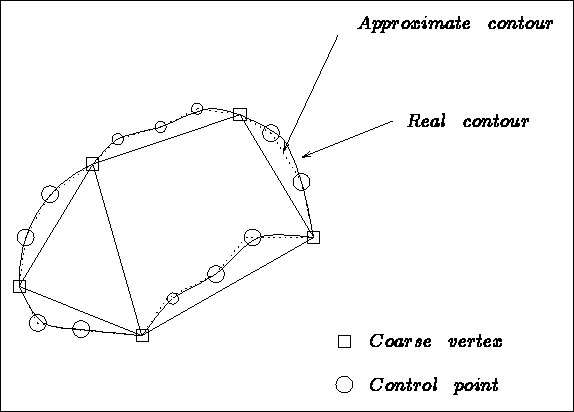Figure 3.1: Domain, initial blocks and approximate domain

Figure 3.1 shows the domain under consideration, the coarse meshing into 2 blocks and its approximation by the given control points. Note that the number of points on the connected sides are the same. Figure 3.2 exhibits the result obtained.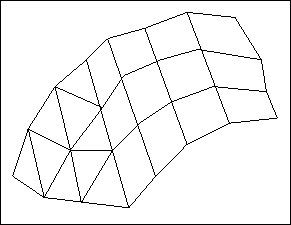Figure 3.2: Mesh of the domain obtained from the description given

The principal steps of the method are given below:

• Data corresponding to the coarse vertices:

The data corresponding to the coarse vertices defines the blocks of the coarse mesh. The latter is input in Rł (to obtain a R˛ mesh we fix side z to the value 0 by indicating that we only want to store the abscissa and ordinates, in the case of blocks of type: segment, triangle and quadrilateral; if not, a surface mesh  is obtained which is coarsely described via these triangular or quadrilateral blocks).

The coarse mesh must fulfill the usual conditions of conformity.

The list of vertices defining the blocks obeys certain rules:

• for a segment or triangle: no order specified
• for a quadrilateral: the first vertex given, input the remaining by traversing the boundary in a given direction (arbitrary)
• for a tetrahedron: no order specified
• for a pentahedron: we input the first triangle (arbitrary order), followed by the second triangle in the same order
• for a hexahedron: we input a quadrilateral face (see the quadrilateral case), followed by the opposite face in the same order

• Data corresponding to the control points on the coarse edges:

The control points enable us to define the real geometry of the block better. They also indicate the number of subdivisions of the splitting to be done. The following rules must by respected:

• for a segment: an arbitrary number of points, n, can be specified
• for a triangle: the same number of points, n, must be specified on each side
• for a quadrilateral: there are 2 numbers to specify: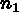points on sides 1 and 3, and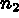points on sides 2 and 4 (these 2 values are independent)
• for a tetrahedron: one single parameter, n, for all the edges
• for a pentahedron: a valueon the edges of the triangular faces and an independent valueon the remaining edges
• for a hexahedron: 3 independent parameters,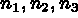, corresponding to the opposite edges
The control points are created automatically or input explicitly.

• Mesh of the edges:

The edges are split into sub-segments joining the control points (created by a module or entered explicitly). A global numbering is allocated to these points.

• Mesh of the faces:

The edge points of the faces are mapped, according to their type, onto a unit triangle or quadrilateral while respecting their respective positions; the unit face is meshed in a canonical fashion and this mesh is projected onto the real face. Let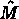be a point in the preceding canonical mesh, with coordinates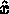and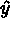, then for a face analogous to a triangle, the following transformation is used: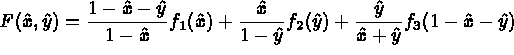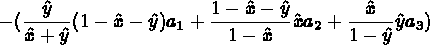where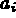designates vertex i of the real face, and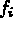is a parametrization of side i of the same face.

In the case of a face analogous to a quadrilateral, the following transformation is used: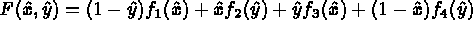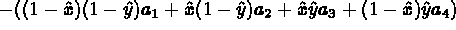using the same notation.

• Splitting of the blocks:

The points obtained at this stage (coarse points, control points of the edges and points on the faces) enable us to apply the same method to blocks. For each block, the collection of these points is projected onto the unit block with corresponding geometry. The latter is meshed in a canonical fashion and then projected onto the real block. The numbers corresponding to the vertices created is constructed with the aid of a global pointer, associated to the triple, i, j, k, giving the position of the point in the block whose value depends on the block treated.

• Final mesh:

The final mesh is obtained as the union of the meshes of the different blocks (the gluing together is automatic as the interfaces (points, edges and common faces of 2 blocks) have been meshed using a global numbering).

The physical attributes of the items created are as follows:

• Point references: the points are either coarse points, or control points on the coarse edges, or coarse face points, or internal points in the blocks. Consequently, reference numbers are those of the coarse points, the coarse edges, the coarse faces, or 0.
• Edge references: the edges are either coarse edges, or edges of a coarse face, or internal edges in the blocks. Consequently, their reference numbers are those of the coarse edges, the coarse faces, or 0.
• Face references: the faces are either supported by a coarse face, or are internal faces in the blocks. Consequently, their reference numbers are those of the coarse faces or 0.
• Element sub-domain numbers: the sub-domain number of the block to which the element belongs is assigned to it.

Remarks:

• Because the blocks have a positive volume, the sub-elements are oriented correctly (the program ensures this correct orientation from the start).
• A mesh created is false if, generally, the geometry of the initial block is too complicated, or if the edge control points are badly positioned.
• The point numbering is in no case optimal (see renumbering method).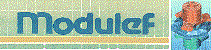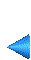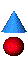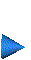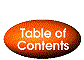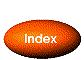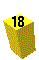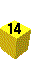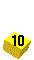Next: 3.2.2 Calling of module COLIB2 Up: 3.2 Multi-block method Prev: 3.2 Multi-block method Index Contents